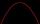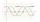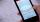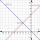# Examples of equations for 9th grade (14y)

#### Number of problems found: 824

• EquationHow many real roots has equation ? ?
• Equation with absSolve this equation with absolute value member: ?Solve quadratic equation: 2x2+28x-550=0
• Reciprocal equationDetermine the root of the equation: 9/x-7/x=1Solve pure quadratic equation ?.Determine the numbers b, c that the numbers x1 = -1 and x2 = 3 were roots of quadratic equation: ?
• Diofant equationIn the set of integers (Z) solve the equation: ? Write result with integer parameter ? (parameter t = ...-2,-1,0,1,2,3... if equation has infinitely many solutions)
• EQ2Solve quadratic equation: ?
• Exponential equationSolve for x: (4^x):0,5=2/64.
• Equation with mixed fractions2 3/5 of 1430+? = 1900. How to do this problem
• SinusDetermine the smallest integer p for which the equation 4 sin x = p has no solution.
• Diofant equation250x + 120y = 5640
• SlopeWhat is the slope of the line defined by the equation -2x +3y = -1 ?
• Diofant 2Is equation ? solvable on the set of integers Z?
• Exponential equationDetermine the value of having y in the expression (3^y): (4^-1)=36. Unknown y is a natural number greater than zero.
• Equation - inverseSolve for x: 7: x = 14: 1000
• Roots countSubstitute the numbers/0,1,2,3/into the equation as x: (x - 1) (x - 3) (x + 1) = 0 Which of them is its solution? Is there another number that solves this equation?
• Eq with reciprocalSolve given equation with reciprocal member: a-6/a+10=4/8
• LineWrite an equation of a line parallel to To 9x + 3y = 8 That Passes Through The Point (-1, -4). Write in form ax+by=c.
• Linear functionWhat is the equation of linear function passing through points: a) A (0,3), B (3,0) b) A (-2,-6), B (3,4)

Do you have an interesting mathematical word problem that you can't solve it? Submit a math problem, and we can try to solve it.

We will send a solution to your e-mail address. Solved examples are also published here. Please enter the e-mail correctly and check whether you don't have a full mailbox.

Please do not submit problems from current active competitions such as Mathematical Olympiad, correspondence seminars etc...

Do you have a linear equation or system of equations and looking for its solution? Or do you have quadratic equation?